## 变换物体与变换坐标系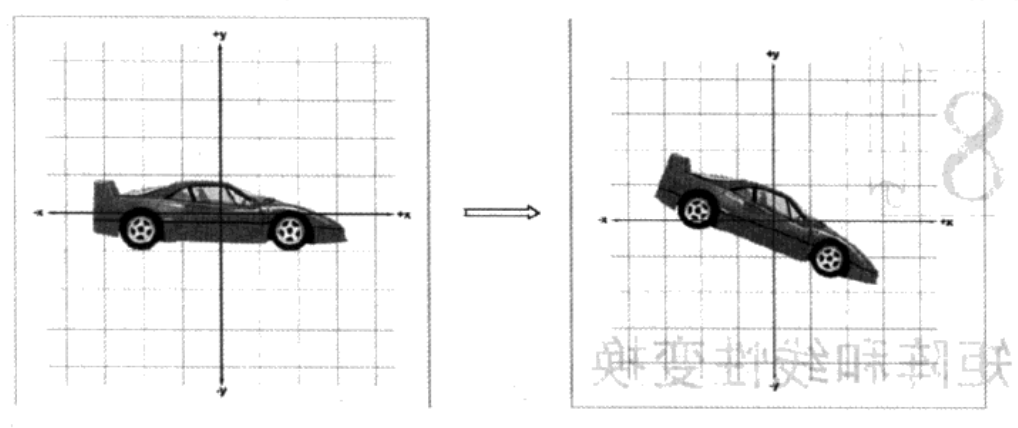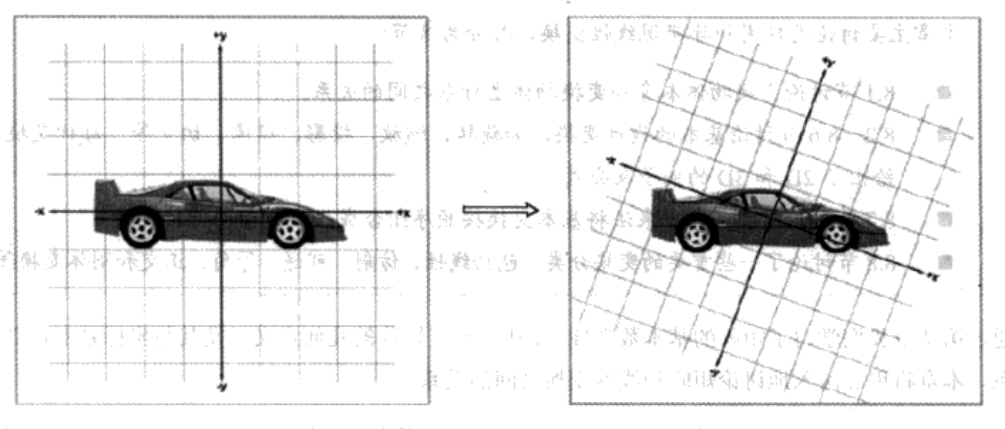那么，为什么还要变换坐标系呢？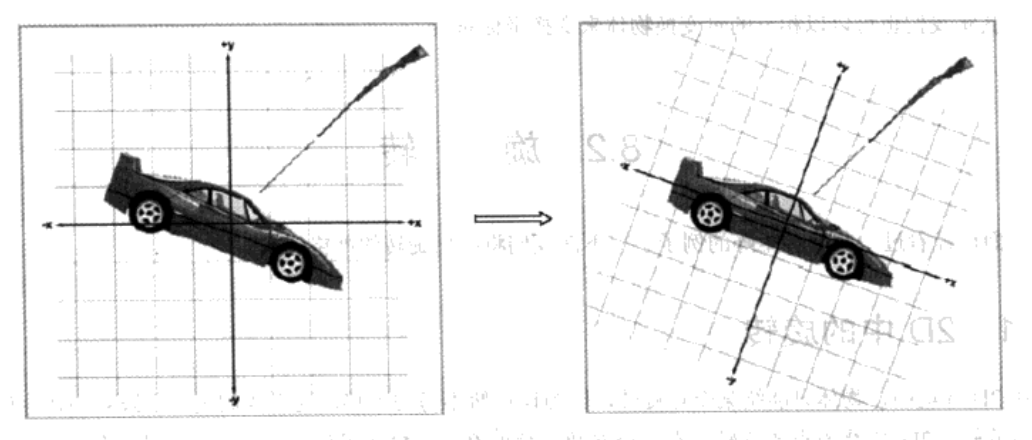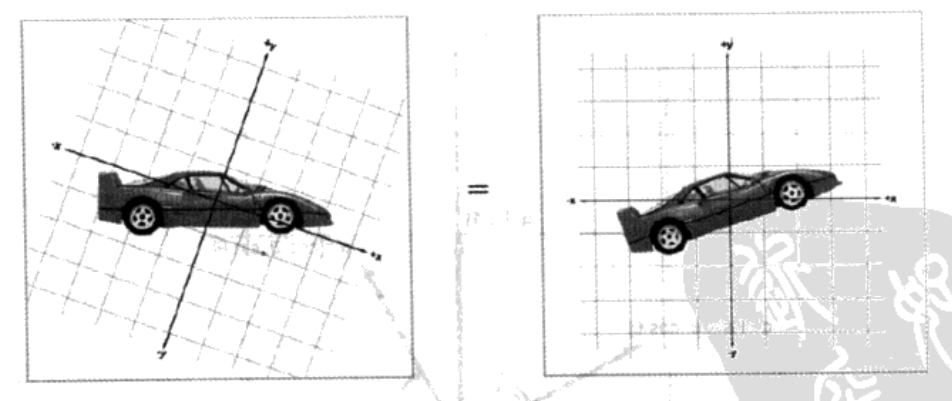## 旋转

### 2D中的旋转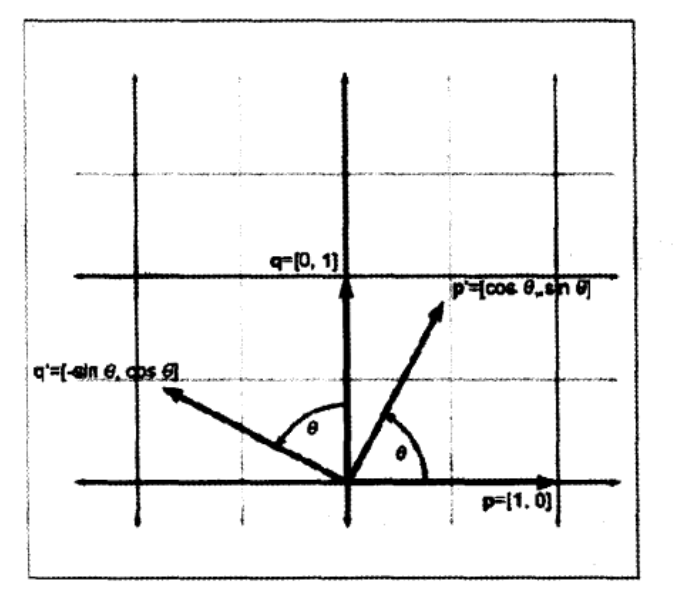$R\left( \theta \right) = \left[ \dfrac {p'} {q'}\right] = \begin{bmatrix} cos\theta & sin\theta \\ -sin\theta & cos \theta \end{bmatrix}$

2D旋转矩阵